# FogLAMP Moving Measure Filter Plugin¶

foglamp-filter-moving-measure performs data smoothing and can be a goto plugin for data-smoothing even with multiple usecases. Additionally, this plugin can be used to calculate measures on sliding window for other purposes too.

Supported combinations are highlighted in the image below.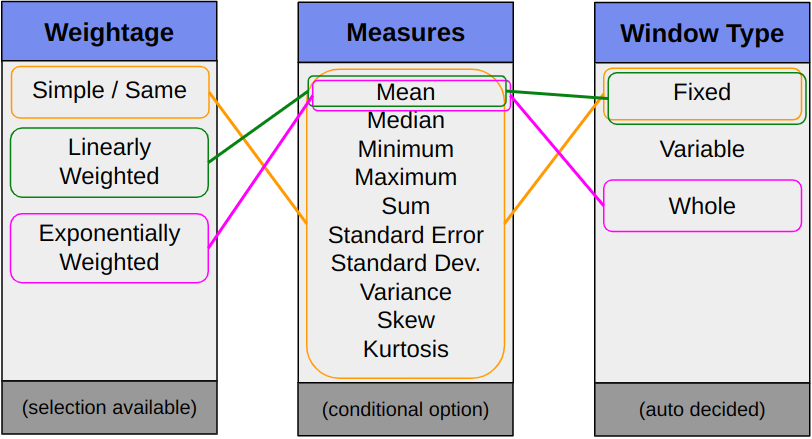## Benefits and Aspects¶

Data Smoothing are data preprocessing techniques to remove noise from the data and to allow important patterns to stand out.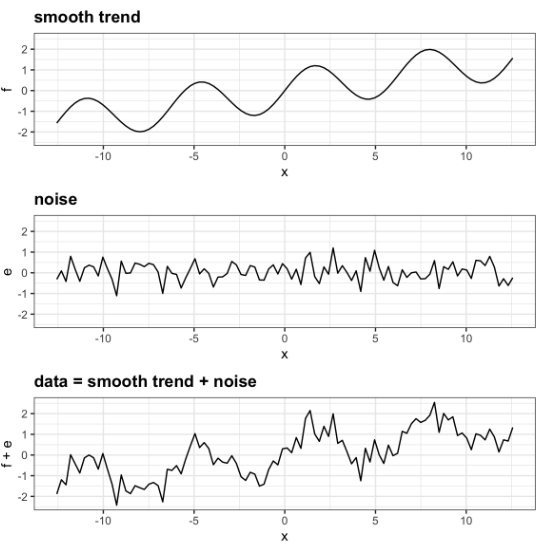When properly applied, smoothing can help us better see patterns, trends, or seasonality. Since smoothing can cause the removal of noises as well as that of outliers and at times even seasonality, hence the data smoothing methodology should be selected with caution or else it’ll even suppress the important signal in the data. In data smoothing there’re multiple techniques and the choice of technique depends on the specific characteristics of the data, the presence of noise, outliers, and the specific objectives of the analysis.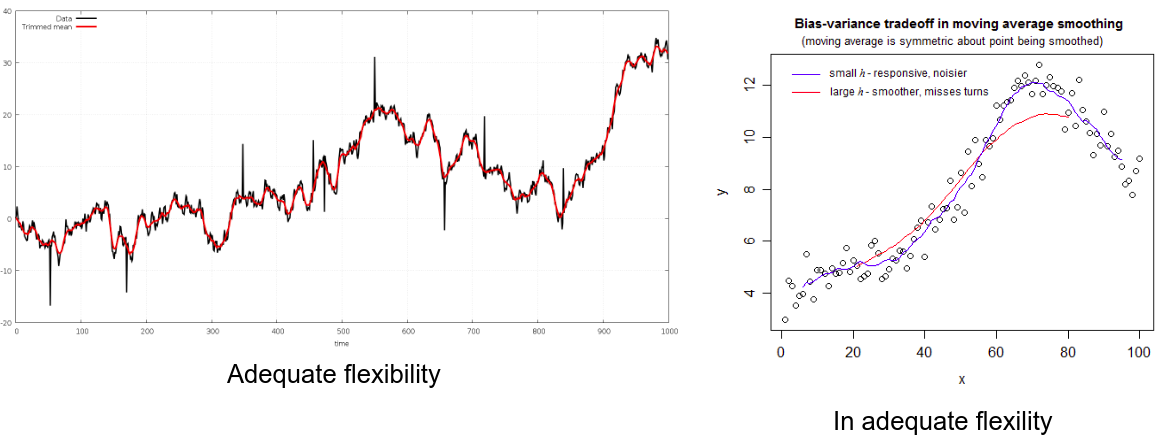### High Level Structure of Plugin¶

This plugin is designed to work with time series data, where time series data is a series of values recorded with respect to a time component (temporal). These plugins will especially be helpful in cases where the values are prone to unnecessary fluctuation maybe because of noise or because it was collected at a very high frequency. At times such an effect can cause the signal or statistics to be lost or make it much more difficult to extract.

There are mainly 3 structural aspects to know while using this plugin. These are mentioned below.

#### 1. Sliding/Moving Window¶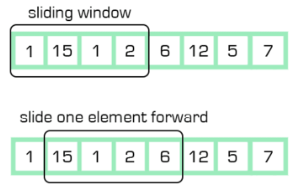This plugin supports the sliding window and this comes by design under a Simple and linearly weighted strategy. Moving window-based aggregates can be applied to smooth time series to handle noise. Over this sliding window of fixed size, some aggregate functions can be applied to get the measure of interest. In a basic sense, it filters out some data from the timeseries wrt to the current time and after a while, it again filters out some data from the now updated timeseries wrt to the now updated current time.

#### 2. Weightage¶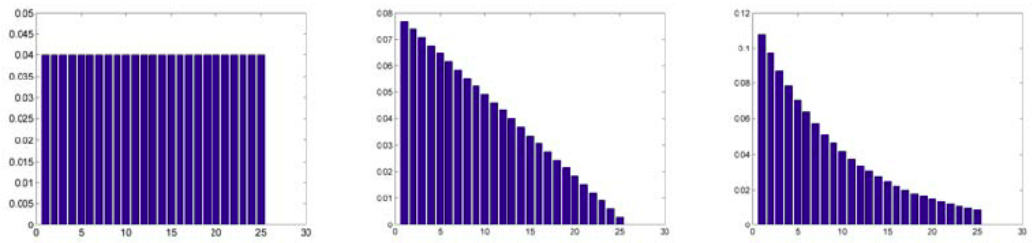Currently, this plugin has support for Simple, Linearly, and Exponential weightage. This weightage in time series data modifies the data wrt to weightage before the aggregation. The reason for the application of weightage is to control the dependence of the measure on recent to past observations. A simple weightage strategy will assign the same with all the data in the window hence there will be a similar dependence on recent data values to that of older data values within the window. While with the exponential weightage strategy, the weightage to the recent observations will be high as compared to older observations.

#### 3. Measure¶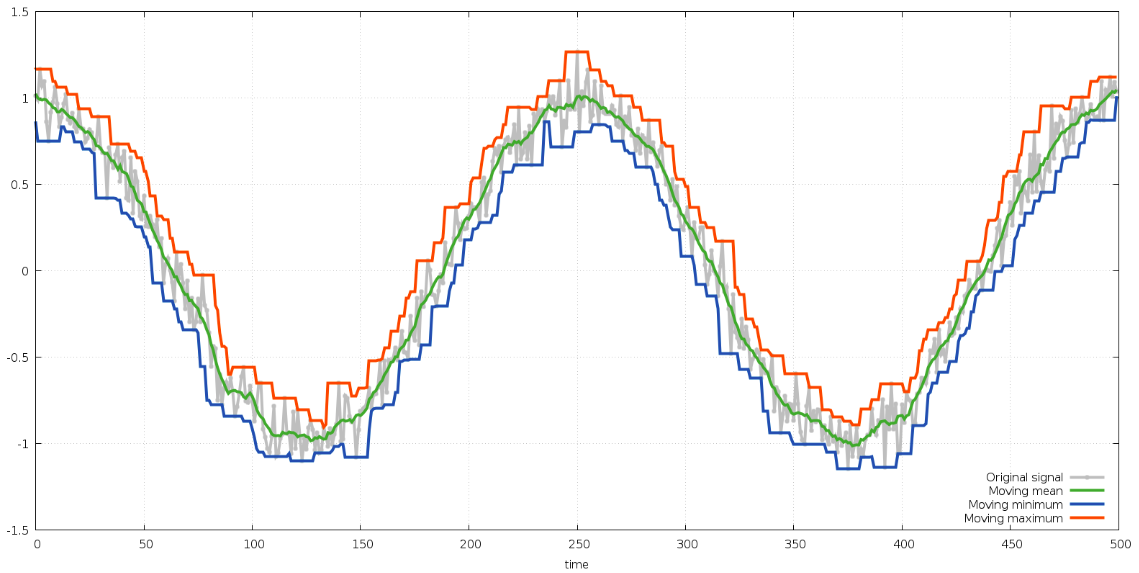These are basically the aggregates or statistics which can be calculated on the weighted values either on the whole series or within the window of mentioned size. The selection and usage of these are based on application or the objective.

Some of the statistics which are covered in this plugin include the following
• Mean

• Median

• Minimum

• Maximum

• Standard Deviation

• Variance

• Standard Error of the Mean

• Sum

• Skew

• Kurtosis

## Configurations¶

Configuring the filter, starts with selecting the filter and adding a service name for it. The screen will look like the following.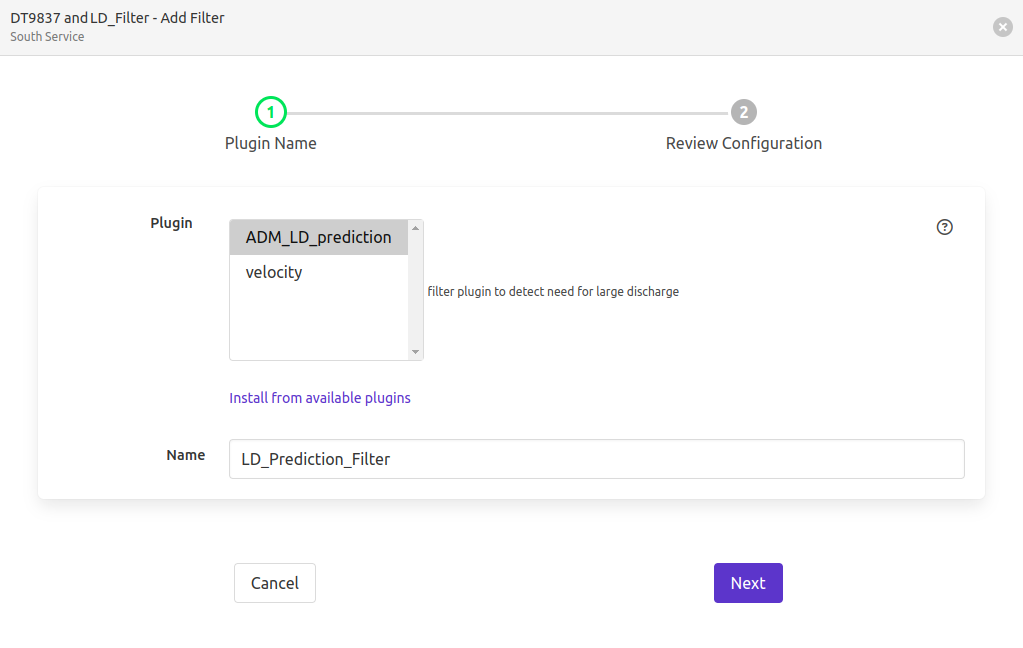Following this the configurations are grouped under 3 sections. Namely,

• Inputs : This will deal with selecting the input time series data

• Strategy : Decide on how to calculate the moving measurements

• Output : Create the suffix for the new datapoint that will be added to the reading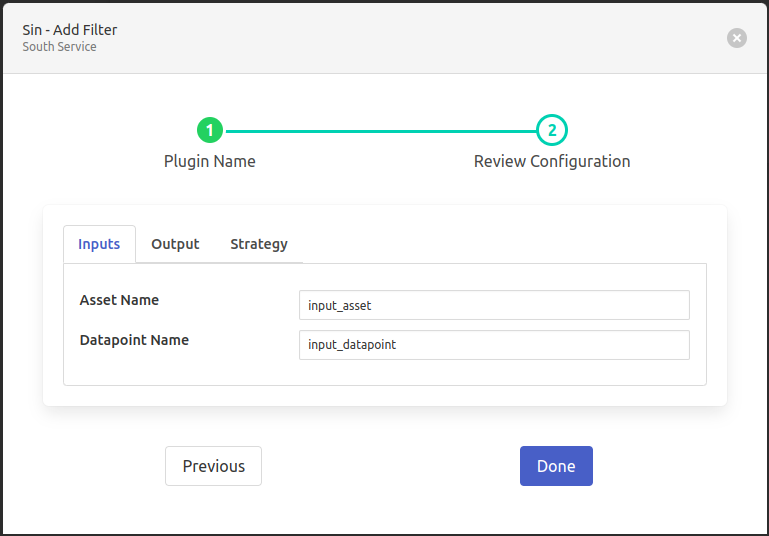• ‘Asset Name’: type: `string` default: ‘input_asset’:

Asset name of the data that will be the input to filter.

• ‘Datapoint Name’: type: `string` default: ‘input_datapoint’:

Asset attribute (datapoint) which will be the input to filter. Note: This must have numeric data.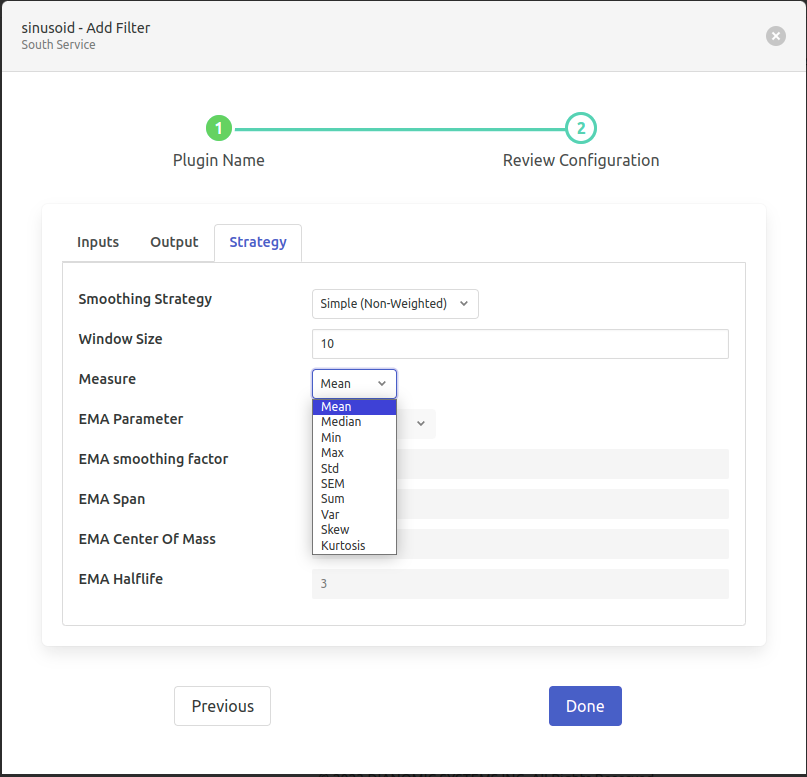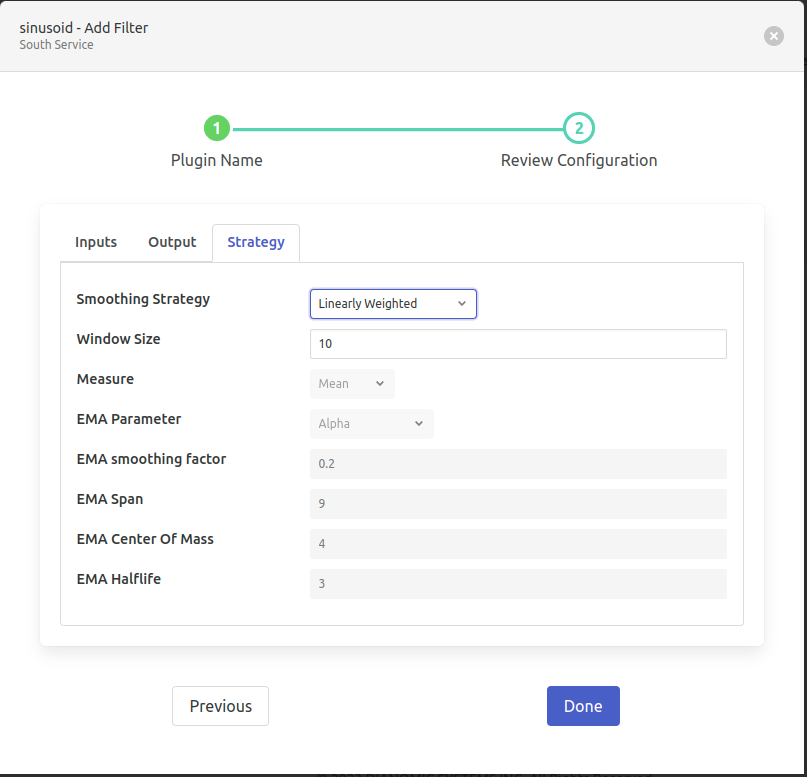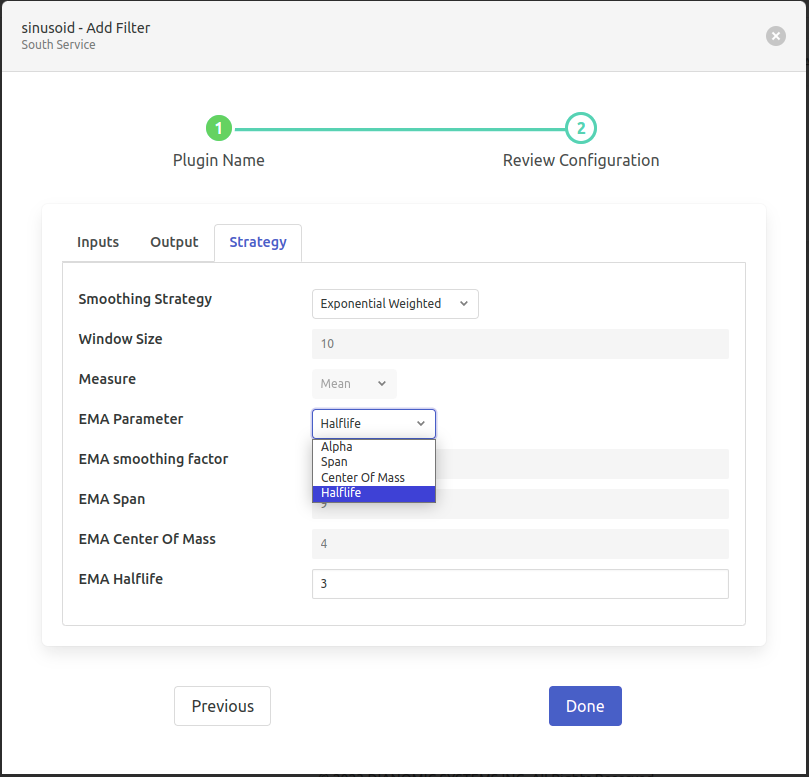• ‘Smoothing Strategy’: type: `enumeration` default: ‘Simple (Non-Weighted)’:

Weighted Strategy for data smoothing. Options

• Simple (Non-Weighted)

• Linearly Weighted

• Exponential Weighted

• ‘Window Size’: type: `integer` default: ‘10’:

Window size that is to be used for Moving Measure calculation

• ‘Measure: type: `enumeration` default: ‘Mean’:

Measure to calculate in the moving fixed size window. Options

• Mean

• Median

• Min

• Max

• Std

• SEM

• Sum

• Var

• Skew

• Kurtosis

• ‘EMA Parameter’: type: `enumeration` default: ‘Alpha’:

The data-smoothing to be performed w.r.t. which parameter. Options

• Alpha

• Span

• Center Of Mass

• Halflife

• ‘EMA Smoothing Factor’: type: `float` default: ‘0.2’:

Specify smoothing factor Range: 0<alpha<=1.

• ‘EMA Span’: type: `float` default: ‘9’:

Specify decay in terms of span. It corresponds to what is commonly called an - N-day Exponential Weighted moving average. Formula: alpha = 2 / (span+1) where span >= 1

• ‘EMA Center Of Mass’: type: `float` default: ‘4’:

Specify decay in terms of center of mass. It has a more physical interpretation and can be thought of in terms of span. Formula: alpha = 1 / (1+c) where com >= 0

• ‘EMA Halflife’: type: `float` default: ‘3’:

Specify decay in terms of half-life. It is the period of time for the exponential weight to reduce to one half. Formula: alpha = 1 - exp**(log 0.5 / halflife) where halflife > 0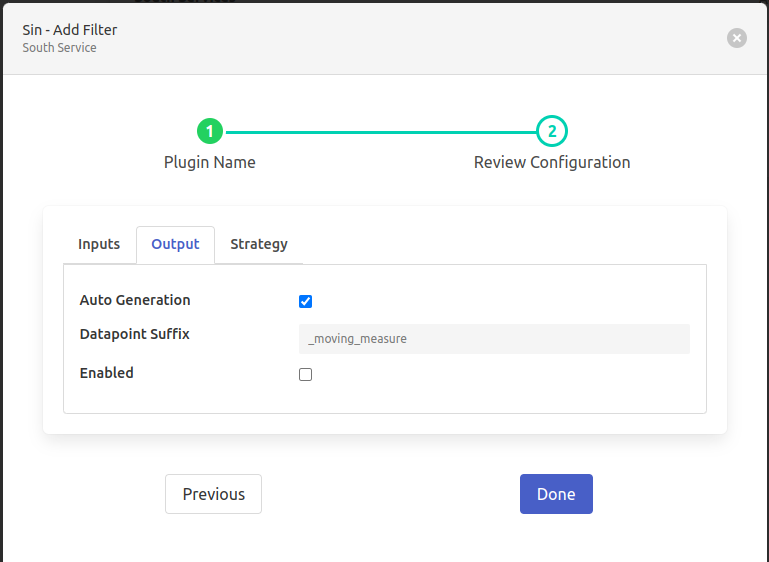• ‘Auto Generation’: type: `boolean` default: ‘true’:

The newly calculated value will be attached with the mentioned input asset. The name of the output datapoint will be the “{input data point}+{output suffix}”. For this output_suffix can be configured by the user. Automatically decide the Output datapoint name based on selected measures “{{Input Datapoint}}{{Output Datapoint Suffix }}” 2nd part will be added.

• ‘Datapoint Suffix’: type: `string` default: ‘_moving_measure’:

Output datapoint name will follow the pattern “{{Input Datapoint}}{{Output Datapoint Suffix }}” 2nd part is customizable.

• ‘Enabled’: type: `boolean` default: ‘false’:

Enable Moving-Measure filter plugin.